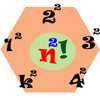Search by Topic

Filter by: Content type:
Age range:
Challenge level:

There are 10 NRICH Mathematical resources connected to Factorials, you may find related items under Numbers and the Number System.

Broad Topics > Numbers and the Number System > FactorialsFac-finding

Age 14 to 16 Challenge Level:

Lyndon chose this as one of his favourite problems. It is accessible but needs some careful analysis of what is included and what is not. A systematic approach is really helpful.Seriesly

Age 16 to 18 Short Challenge Level:

Prove that k.k! = (k+1)! - k! and sum the series 1.1! + 2.2! + 3.3! +...+n.n!How Does Your Function Grow?

Age 16 to 18 Challenge Level:

Compares the size of functions f(n) for large values of n.The Harmonic Triangle and Pascal's Triangle

Age 16 to 18

The harmonic triangle is built from fractions with unit numerators using a rule very similar to Pascal's triangle.Factorial Fun

Age 16 to 18 Challenge Level:

How many divisors does factorial n (n!) have?Factorial

Age 14 to 16 Challenge Level:

How many zeros are there at the end of the number which is the product of first hundred positive integers?Seriesly

Age 16 to 18 Challenge Level:

Prove that k.k! = (k+1)! - k! and sum the series 1.1! + 2.2! + 3.3! +...+n.n!Squaresearch

Age 14 to 16 Challenge Level:

Consider numbers of the form un = 1! + 2! + 3! +...+n!. How many such numbers are perfect squares?N000ughty Thoughts

Age 14 to 16 Challenge Level:

How many noughts are at the end of these giant numbers?Growing

Age 16 to 18 Challenge Level:

Which is larger: (a) 1.000001^{1000000} or 2? (b) 100^{300} or 300! (i.e.factorial 300)